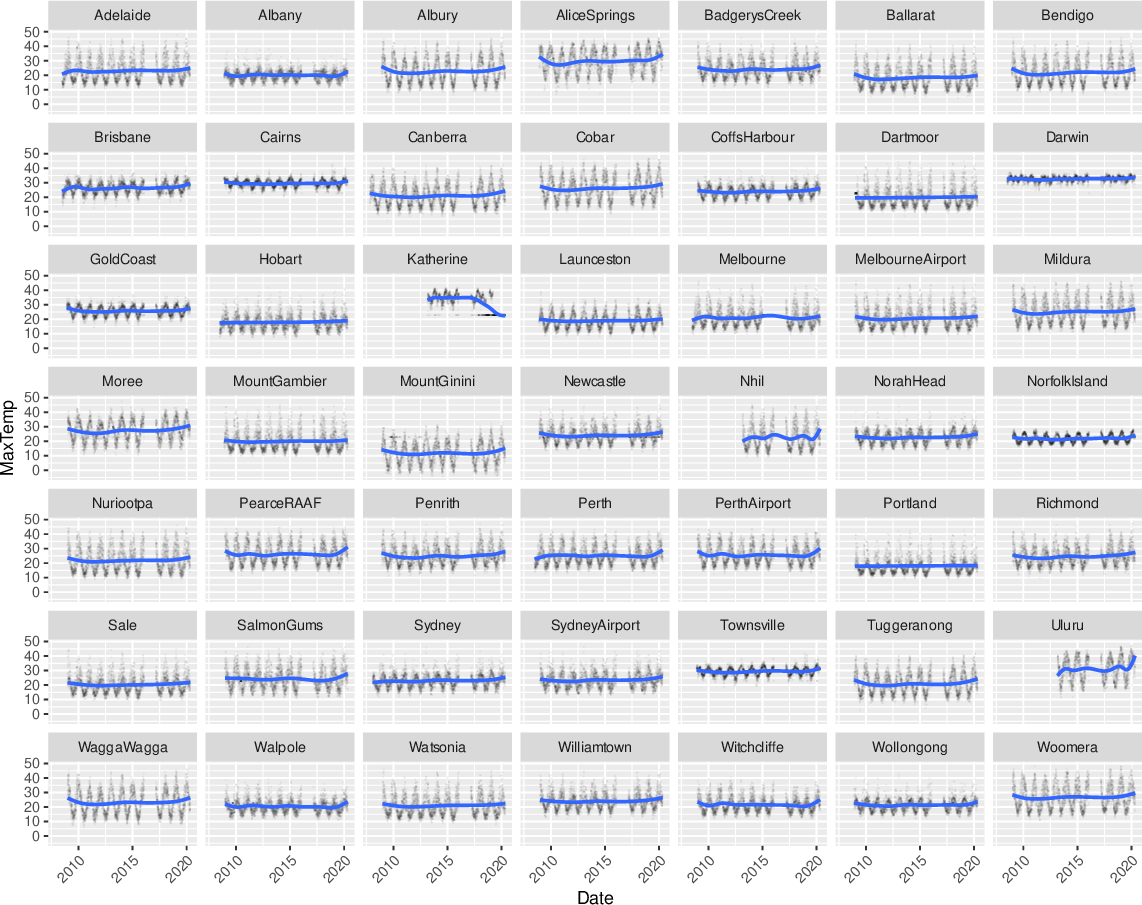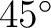Data Science Desktop Survival Guide by Graham WilliamsDesktop Survival Project Home Preface Data Science Introducing R R Constructs R Tasks R Strings R Read, Write, and Create Data Template Data Exploration Data Wrangling Data Visualisation Statistics ML Template ML Scenarios ML Activities ML Applications ML Algorithms Cluster Analysis Decision Trees Computer Vision Graph Data Privacy Literate Data Science Coding with Style Resources Bibliography Index

## Faceted Location Scatter Plot

Rawds %>%   ggplot(aes(x=date, y=max_temp)) +   geom_point(alpha=0.05, shape=".") +   geom_smooth(method="gam", formula=y~s(x, bs="cs")) +   facet_wrap(~location) +   theme(axis.text.x=element_text(angle=45, hjust=1)) +   labs(x=vnames["date"], y=vnames["max_temp"]) Partitioning the dataset by a categoric variable reduces the blob effect for big data. The plot uses location as the faceted variable to separately plot each location's maximum temperature over time. Notice the seasonal effect across all plots, some with quite different patterns. The plot uses facet_wrap() to separately plot each location. Using ggplot2::geom_point() with alpha= reduces the effect of overlaid points as does using smaller dots on the plots by way of shape=. Together this works to de-clutter the plot and improves the presentation with an emphasis on the patterns. The x-axis tick labels are rotatedusing angle=45 within ggplot2::element_text() to avoid the labels overlapping. The hjust=1 forces the labels to be right justified.